Скачать презентацию 1 9 2001 FINANCIAL ENGINEERING DERIVATIVES AND RISK MANAGEMENT J

f2f7a362acef35c93a2344a12743ba4f.ppt

• Количество слайдов: 18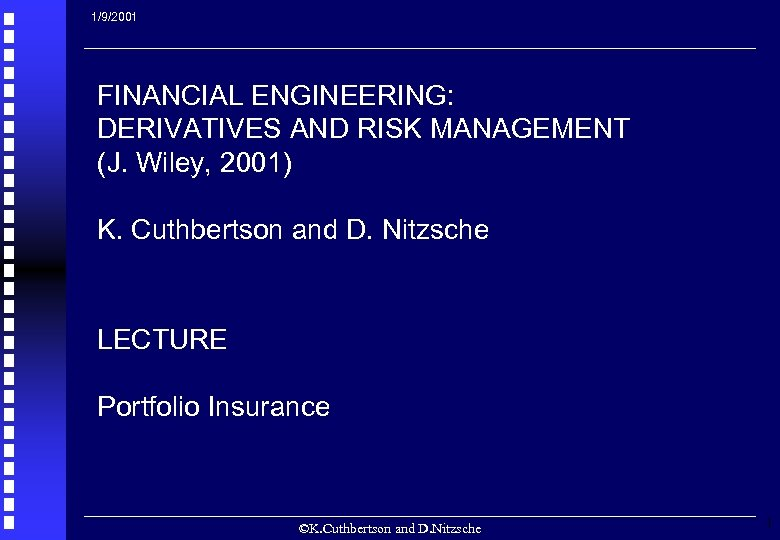1/9/2001 FINANCIAL ENGINEERING: DERIVATIVES AND RISK MANAGEMENT (J. Wiley, 2001) K. Cuthbertson and D. Nitzsche LECTURE Portfolio Insurance ©K. Cuthbertson and D. Nitzsche 1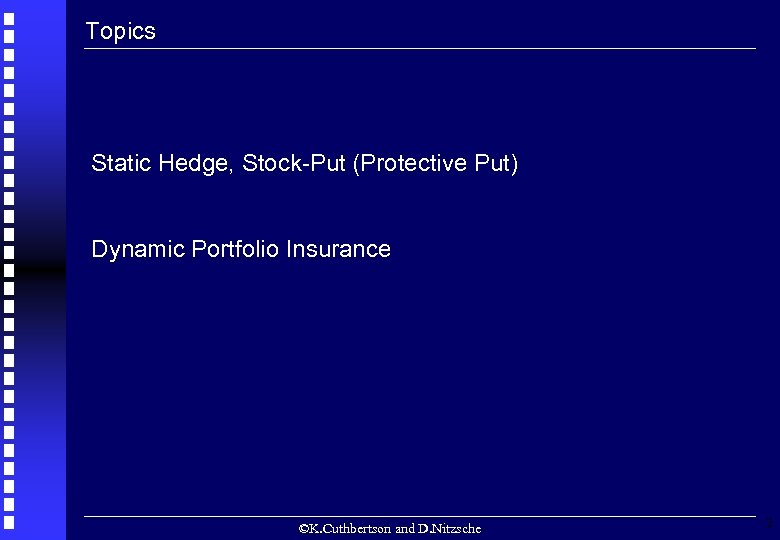Topics Static Hedge, Stock-Put (Protective Put) Dynamic Portfolio Insurance ©K. Cuthbertson and D. Nitzsche 2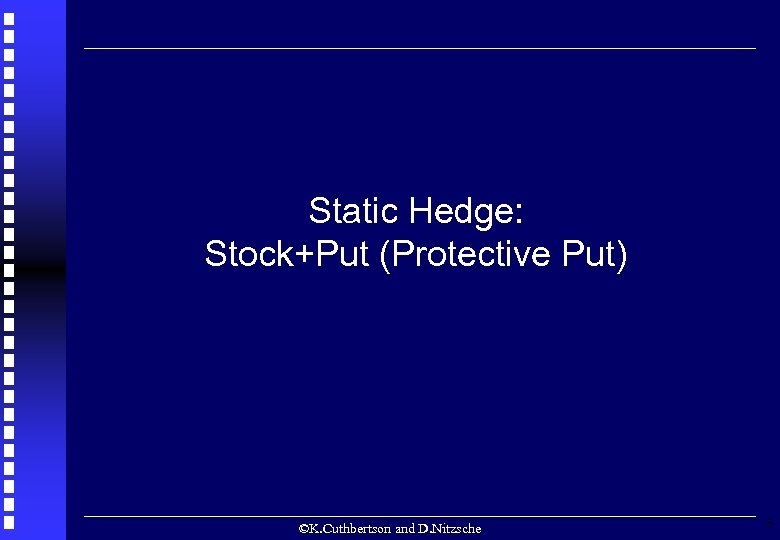Static Hedge: Stock+Put (Protective Put) ©K. Cuthbertson and D. Nitzsche 3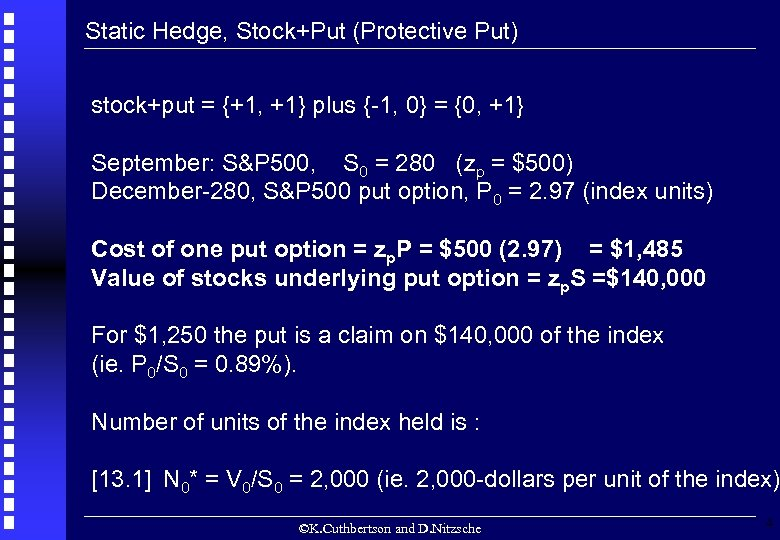Static Hedge, Stock+Put (Protective Put) stock+put = {+1, +1} plus {-1, 0} = {0, +1} September: S&P 500, S 0 = 280 (zp = \$500) December-280, S&P 500 put option, P 0 = 2. 97 (index units) Cost of one put option = zp. P = \$500 (2. 97) = \$1, 485 Value of stocks underlying put option = zp. S =\$140, 000 For \$1, 250 the put is a claim on \$140, 000 of the index (ie. P 0/S 0 = 0. 89%). Number of units of the index held is : [13. 1] N 0* = V 0/S 0 = 2, 000 (ie. 2, 000 -dollars per unit of the index) ©K. Cuthbertson and D. Nitzsche 4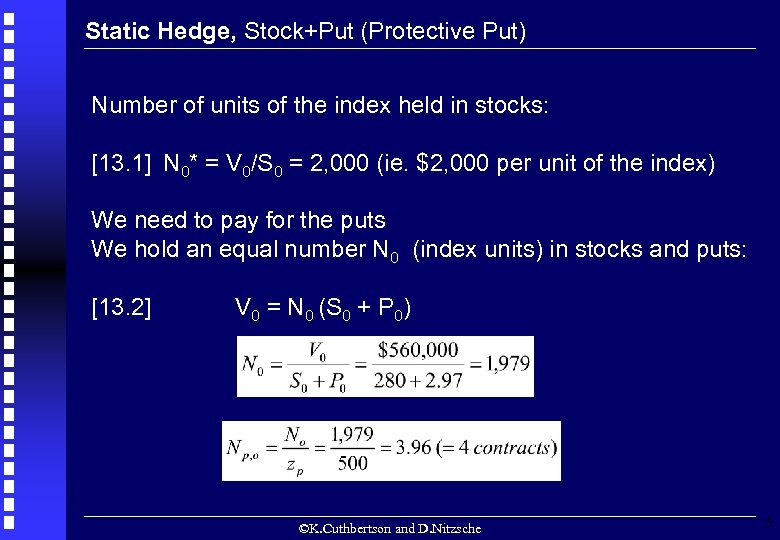Static Hedge, Stock+Put (Protective Put) Number of units of the index held in stocks: [13. 1] N 0* = V 0/S 0 = 2, 000 (ie. \$2, 000 per unit of the index) We need to pay for the puts We hold an equal number N 0 (index units) in stocks and puts: [13. 2] V 0 = N 0 (S 0 + P 0) ©K. Cuthbertson and D. Nitzsche 5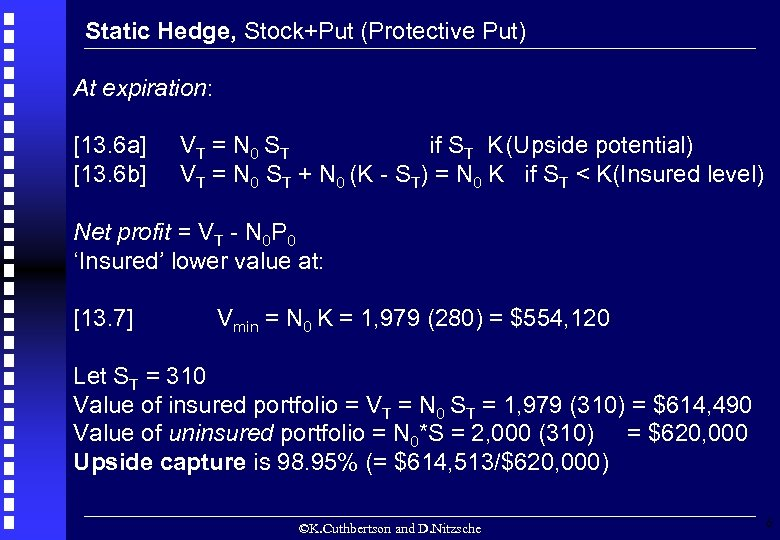Static Hedge, Stock+Put (Protective Put) At expiration: [13. 6 a] [13. 6 b] VT = N 0 ST if ST K(Upside potential) VT = N 0 ST + N 0 (K - ST) = N 0 K if ST < K(Insured level) Net profit = VT - N 0 P 0 ‘Insured’ lower value at: [13. 7] Vmin = N 0 K = 1, 979 (280) = \$554, 120 Let ST = 310 Value of insured portfolio = VT = N 0 ST = 1, 979 (310) = \$614, 490 Value of uninsured portfolio = N 0*S = 2, 000 (310) = \$620, 000 Upside capture is 98. 95% (= \$614, 513/\$620, 000) ©K. Cuthbertson and D. Nitzsche 6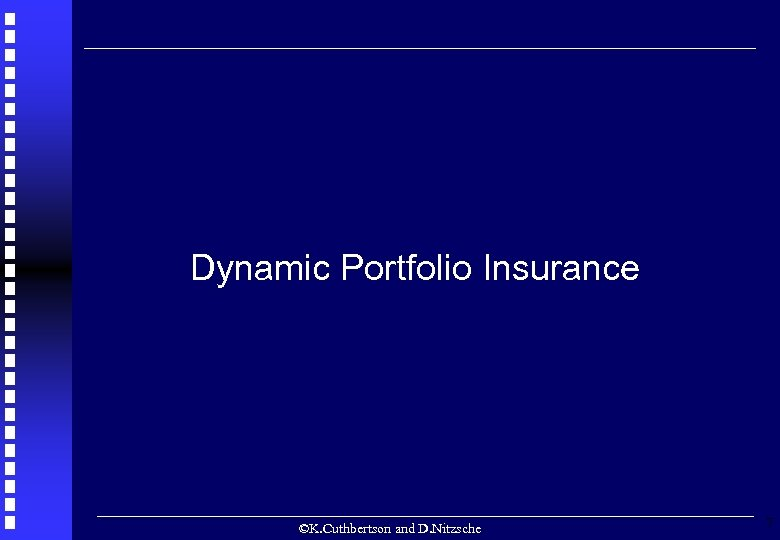Dynamic Portfolio Insurance ©K. Cuthbertson and D. Nitzsche 7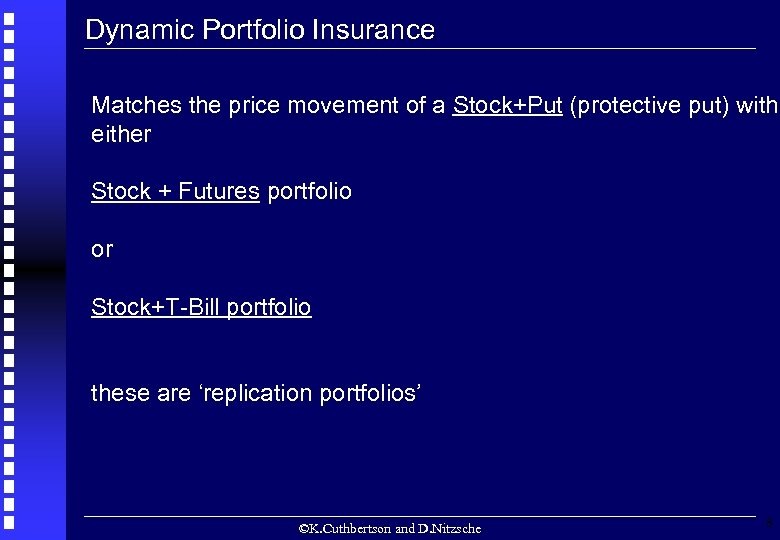Dynamic Portfolio Insurance Matches the price movement of a Stock+Put (protective put) with either Stock + Futures portfolio or Stock+T-Bill portfolio these are ‘replication portfolios’ ©K. Cuthbertson and D. Nitzsche 8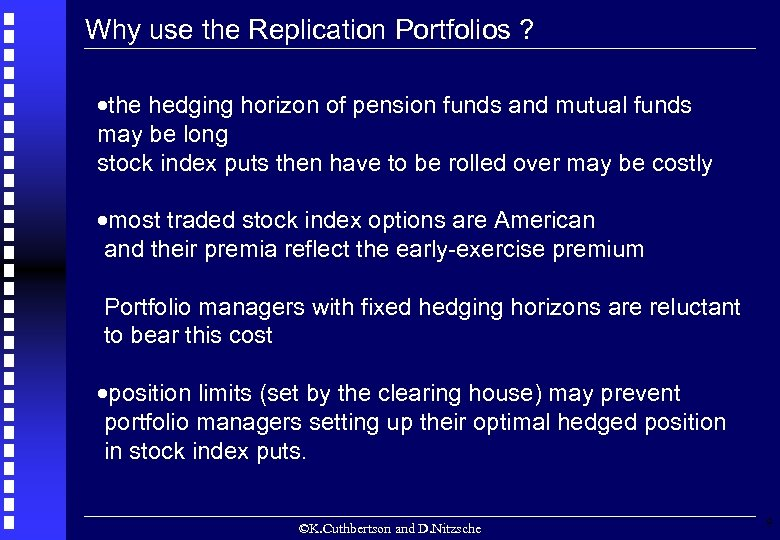Why use the Replication Portfolios ? ·the hedging horizon of pension funds and mutual funds may be long stock index puts then have to be rolled over may be costly ·most traded stock index options are American and their premia reflect the early-exercise premium Portfolio managers with fixed hedging horizons are reluctant to bear this cost ·position limits (set by the clearing house) may prevent portfolio managers setting up their optimal hedged position in stock index puts. ©K. Cuthbertson and D. Nitzsche 9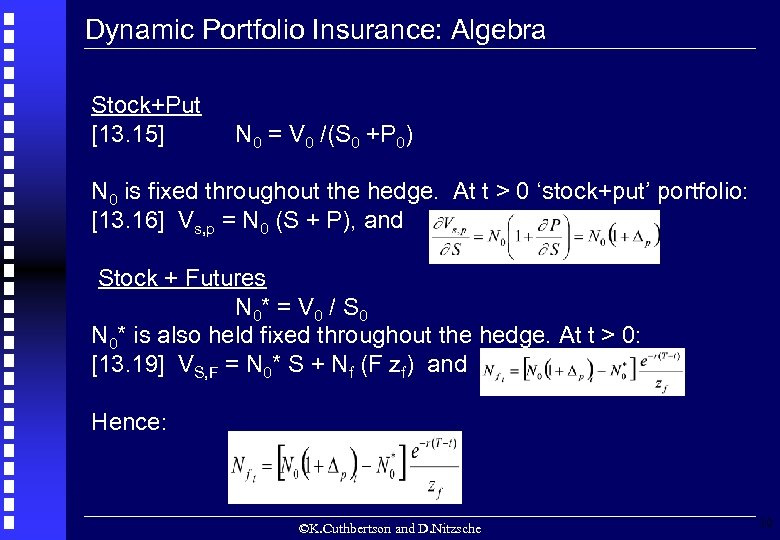Dynamic Portfolio Insurance: Algebra Stock+Put [13. 15] N 0 = V 0 /(S 0 +P 0) N 0 is fixed throughout the hedge. At t > 0 ‘stock+put’ portfolio: [13. 16] Vs, p = N 0 (S + P), and Stock + Futures N 0* = V 0 / S 0 N 0* is also held fixed throughout the hedge. At t > 0: [13. 19] VS, F = N 0* S + Nf (F zf) and Hence: ©K. Cuthbertson and D. Nitzsche 10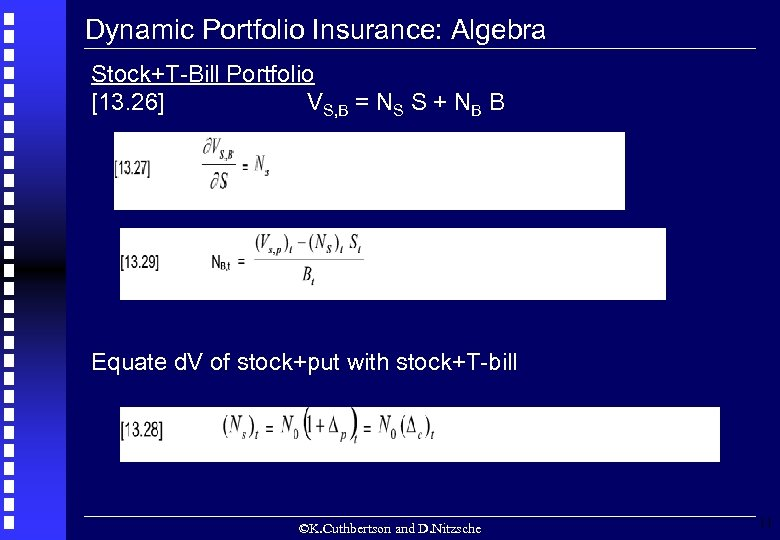Dynamic Portfolio Insurance: Algebra Stock+T-Bill Portfolio [13. 26] VS, B = NS S + NB B Equate d. V of stock+put with stock+T-bill ©K. Cuthbertson and D. Nitzsche 11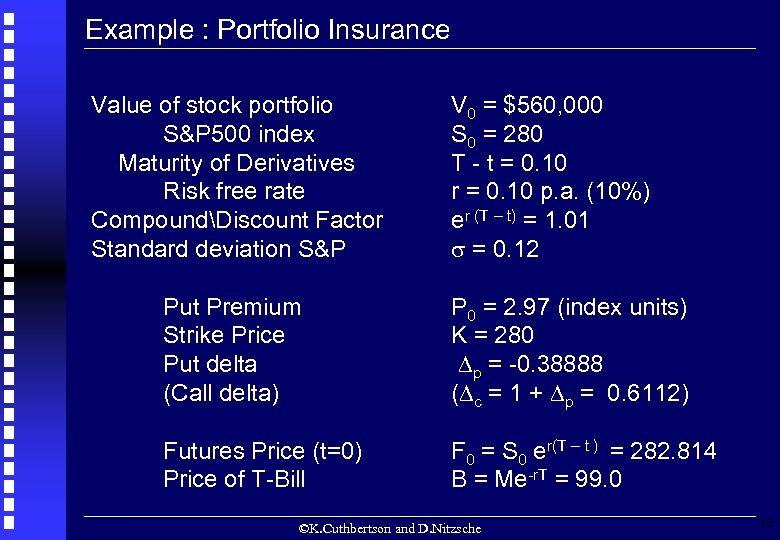Example : Portfolio Insurance Value of stock portfolio S&P 500 index Maturity of Derivatives Risk free rate CompoundDiscount Factor Standard deviation S&P V 0 = \$560, 000 S 0 = 280 T - t = 0. 10 r = 0. 10 p. a. (10%) er (T – t) = 1. 01 = 0. 12 Put Premium Strike Price Put delta (Call delta) P 0 = 2. 97 (index units) K = 280 p = -0. 38888 ( c = 1 + p = 0. 6112) Futures Price (t=0) Price of T-Bill F 0 = S 0 er(T – t ) = 282. 814 B = Me-r. T = 99. 0 ©K. Cuthbertson and D. Nitzsche 12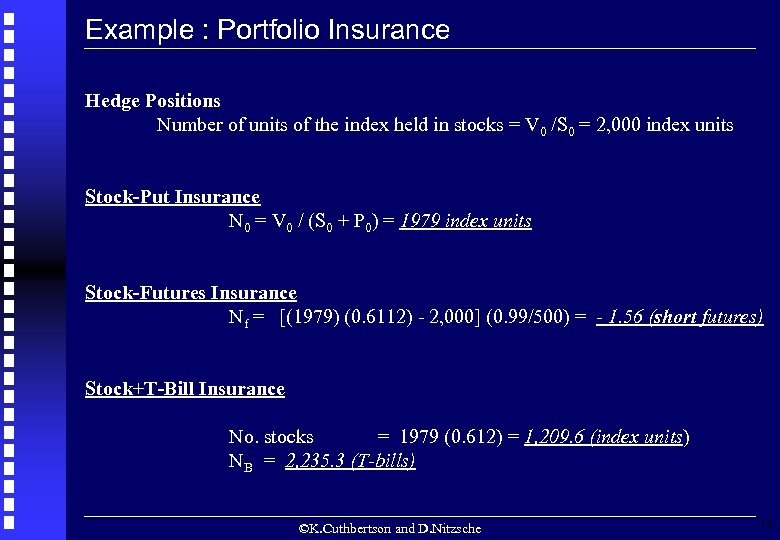Example : Portfolio Insurance Hedge Positions Number of units of the index held in stocks = V 0 /S 0 = 2, 000 index units Stock-Put Insurance N 0 = V 0 / (S 0 + P 0) = 1979 index units Stock-Futures Insurance Nf = [(1979) (0. 6112) - 2, 000] (0. 99/500) = - 1. 56 (short futures) Stock+T-Bill Insurance No. stocks = 1979 (0. 612) = 1, 209. 6 (index units) NB = 2, 235. 3 (T-bills) ©K. Cuthbertson and D. Nitzsche 13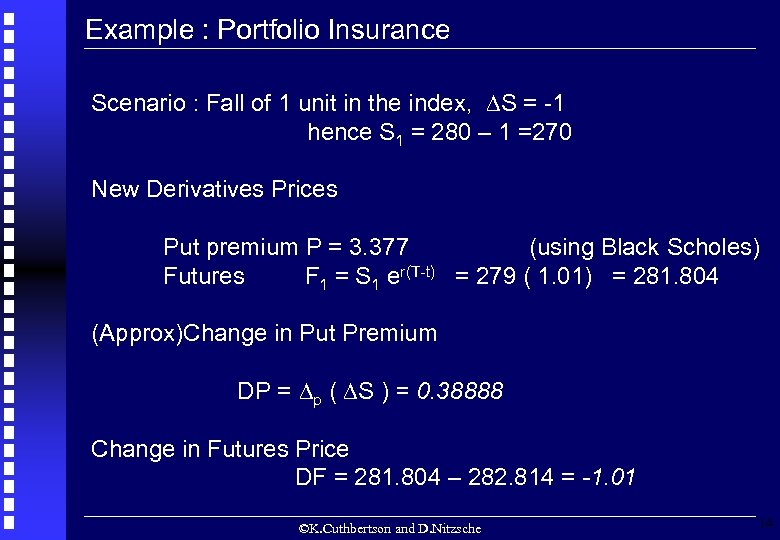Example : Portfolio Insurance Scenario : Fall of 1 unit in the index, S = -1 hence S 1 = 280 – 1 =270 New Derivatives Prices Put premium P = 3. 377 (using Black Scholes) Futures F 1 = S 1 er(T-t) = 279 ( 1. 01) = 281. 804 (Approx)Change in Put Premium DP = p ( S ) = 0. 38888 Change in Futures Price DF = 281. 804 – 282. 814 = -1. 01 ©K. Cuthbertson and D. Nitzsche 14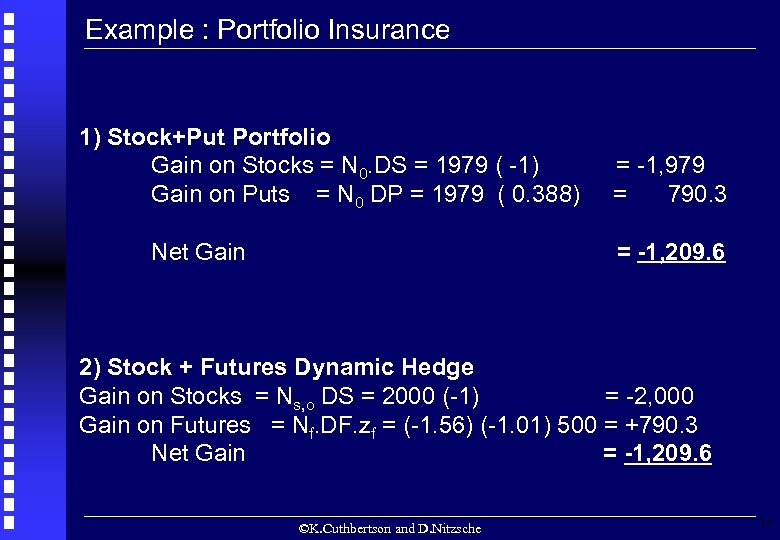Example : Portfolio Insurance 1) Stock+Put Portfolio Gain on Stocks = N 0. DS = 1979 ( -1) Gain on Puts = N 0 DP = 1979 ( 0. 388) Net Gain = -1, 979 = 790. 3 = -1, 209. 6 2) Stock + Futures Dynamic Hedge Gain on Stocks = Ns, o DS = 2000 (-1) = -2, 000 Gain on Futures = Nf. DF. zf = (-1. 56) (-1. 01) 500 = +790. 3 Net Gain = -1, 209. 6 ©K. Cuthbertson and D. Nitzsche 15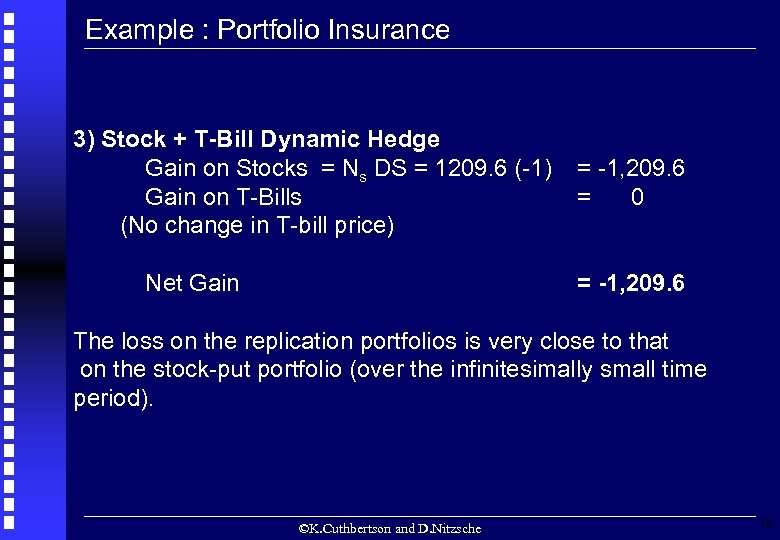Example : Portfolio Insurance 3) Stock + T-Bill Dynamic Hedge Gain on Stocks = Ns DS = 1209. 6 (-1) Gain on T-Bills (No change in T-bill price) Net Gain = -1, 209. 6 = 0 = -1, 209. 6 The loss on the replication portfolios is very close to that on the stock-put portfolio (over the infinitesimally small time period). ©K. Cuthbertson and D. Nitzsche 16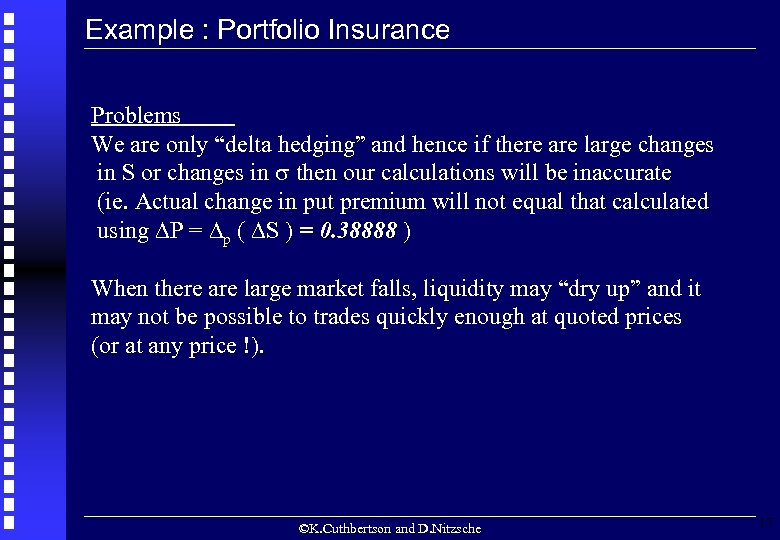Example : Portfolio Insurance Problems We are only “delta hedging” and hence if there are large changes in S or changes in then our calculations will be inaccurate (ie. Actual change in put premium will not equal that calculated using P = p ( S ) = 0. 38888 ) When there are large market falls, liquidity may “dry up” and it may not be possible to trades quickly enough at quoted prices (or at any price !). ©K. Cuthbertson and D. Nitzsche 17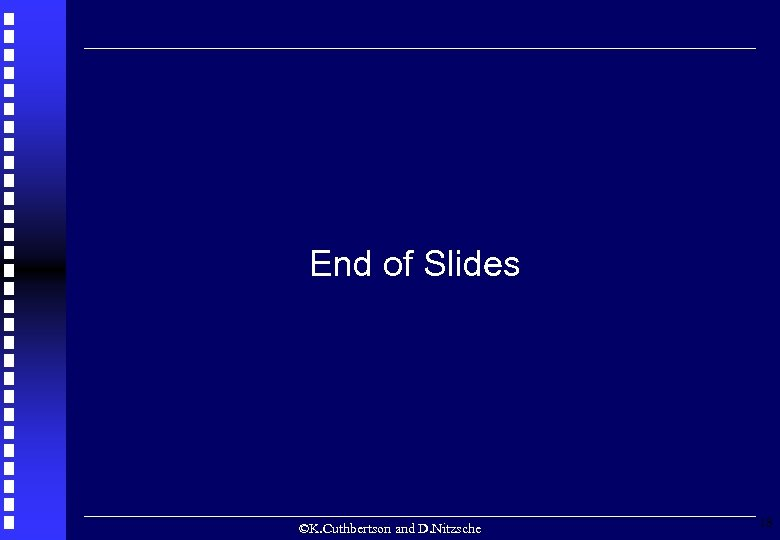End of Slides ©K. Cuthbertson and D. Nitzsche 18